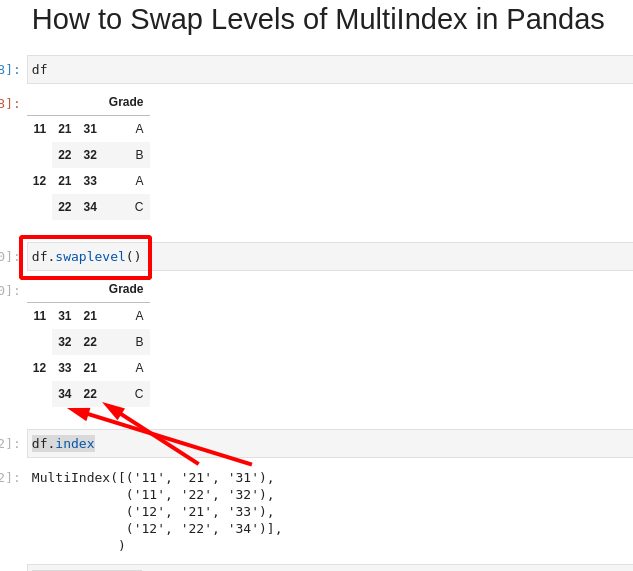In this short guide, I'll show you how to swap levels of MultiIndex in Pandas DataFrame.

You can also find how to reorder MultiIndex from left to right(and reverse), swap multiple levels and typical errors.

So at the end we will get different order of the MultiIndex levels from:

``````MultiIndex([('11', '21', '31'),
('11', '22', '32'),
('12', '21', '33'),
('12', '22', '34')],
)
``````

to:

``````MultiIndex([('11', '31', '21'),
('11', '32', '22'),
('12', '33', '21'),
('12', '34', '22')],
)
``````

Notice that the inner levels changed their positions. This is done by method:

``````df.swaplevel()
``````

Let's cover the MultiIndex reorder in more detail.

## Setup

To start let's create a simple DataFrame with MultiIndex:

``````import pandas as pd

df = pd.DataFrame(
index=[
["11", "11", "12", "12"],
["21", "22", "21", "22"],
["31", "32", "33", "34"]
]
)
``````

The resulted DataFrame is:

11 21 31 A
22 32 B
12 21 33 A
22 34 C

We can get the index by:

``````df.index
``````

result is a MultiIndex:

``````MultiIndex([('11', '21', '31'),
('11', '22', '32'),
('12', '21', '33'),
('12', '22', '34')],
)
``````

## Step 1: Method swaplevel()

We can use the method `swaplevel()` to swap levels of DataFrame with MultiIndex.

The method's documentation is available from: DataFrame.swaplevel.

The method signature is:

``````DataFrame.swaplevel(i=- 2, j=- 1, axis=0)
``````

Where the parameters are:

• `i, j` - Levels of the indices to be swapped (int or str)
• `axis` - 0 or `index`, 1 or `columns` - column-wise

We covered the result of the default behavior above. Here we will explain it.

Default behavior

``````df.swaplevel()
``````

is equivalent of:

``````df.swaplevel(i=-2, j=-1, axis=0)
``````

So it will swap the two innermost levels row-wise.## Step 2: Swap Levels of MultiIndex - column-wise

Swapping levels of MultiIndex column-wise is possible by using parameter `axis=1`.

``````df.swaplevel(axis=1)
``````

## Step 3: Swap Outer Levels of MultiIndex

Levels of the MultiIndex:

``````MultiIndex([('11', '21', '31'),
('11', '22', '32'),
('12', '21', '33'),
('12', '22', '34')],
)
``````

Are numbered as:

• 0
• 1
• 2

To get level 0 we can do:

``````df.index.get_level_values(0)
``````

The result is index:

``````Index(['11', '11', '12', '12'], dtype='object')
``````

So to swap the levels from the left to the right we can use positive numbers:

``````df.swaplevel(i=0, j=1)
``````

From right to the left we can pass negative indices:

``````df.swaplevel(i=-1, j=-2)
``````
× Pro Tip 1
`df.swaplevel(i=-1, j=-2)`
is equivalent to
`df.swaplevel(i=-2, j=-1)`

## Step 4: reorder_levels() - swap multiple levels

Method `reorder_levels()` can be used to swap multiple levels of MultiIndex at once.

It requires the level order as parameter:

``````df.reorder_levels([1,2,0]).index
``````

result:

``````MultiIndex([('21', '31', '11'),
('22', '32', '11'),
('21', '33', '12'),
('22', '34', '12')],
)
``````

## Step 5: TypeError: Can only swap levels on a hierarchical axis.

If you get error like:

TypeError: Can only swap levels on a hierarchical axis.

It means that you are trying to use the method `swaplevel()` on a flat index. You need to check what is the index by:

``````df.index
``````

## Step 6: IndexError: Too many levels: Index has only 3 levels, not 4

If we try to pass higher level than the one existing on the MultiIndex we will get an error:

IndexError: Too many levels: Index has only 3 levels, not 4

We need to check the levels with:

``````df.index.names
len(df.index.names)
``````

This will give us the names of the levels( and the number):

``````FrozenList([None, None, None])
3
``````

or

``````df.index.shape
``````

This will give us:

``````(4,)
``````

Which is the number of the rows.

## Conclusion

This article covers how to swap levels of MultiIndex. It shows different options and most common problems.

It gives hints on how to deal with MultiIndex and how to get information about the levels.